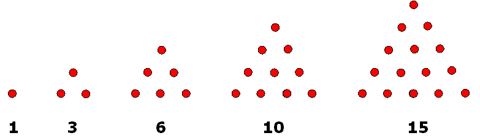Home > Patterns > Misunderstandings > Number sequences

# Number sequences

Students may start learning about repeating patterns as early as preschool. They learn that, in such patterns, the unit of repeat is fixed.

The next stage is to learn that many patterns (called growing patterns) do not have a fixed unit of repeat. They still have 'chunks', called terms, but these terms change in some systematic way. The terms of a growing pattern may involve number, direction, shape, size — almost anything imaginable.

An important type of growing pattern is the number sequence. Because mathematical patterns are regular, every number sequence must have at least one rule that explains how each term is obtained.

This rule may explain how each term is obtained from the previous term. For example, in the number sequence 1, 3, 6, 10, 15… each term is obtained by adding successive whole numbers to the previous term (i.e. 3 = 1 + 2, 6 = 3 + 3, 10 = 6 + 4, etc.).

In other cases, the rule may explain each individual term separately. For example, each number in the sequence 1, 3, 6, 10, 15… gives the number of dots in a growing pattern of triangular arrays. In this growing pattern, you can also see that the number of dots increases by successive whole numbers.Triangular numbers.

It is often difficult for students to find rules for number sequences.

## Introducing number sequences

Number sequences can be introduced via repeating patterns. It is important to progress from concrete materials to pictures and drawings before introducing abstract number patterns.

## Let's have a party!

Here is an example of how an understanding of growing patterns can help students solve problems.

## Find a rule

Here is an example of a growing pattern that can be difficult for students to continue.